# Theory of Simple BendingReading time: 1 minute

When a beam is subjected to a loading system or by a force couple acting on a plane passing through the axis, then the beam deforms. In simple terms, this axial deformation is called as bending of a beam. Due to the shear force and bending moment, the beam undergoes deformation. These normal stress due to bending are called flexure stresses.Fig 1: Types of bending stress in a beam section

## Assumptions to calculate bending stress

These stresses formed in the  material due to bending can be calculated using certian assumption, they are
1. Beam is initially straight , and has a constant cross-section.
2. Beam is made of homogeneous material and the beam has a longitudinal plane of symmetry.
3. Resultant of the applied loads lies in the plane of symmetry.
4. The geometry of the overall member is such that bending not buckling is the primary cause of failure.
5. Elastic limit is nowhere exceeded and ‘E' is same in tension and compression.
6. Plane cross - sections remains plane before and after bending.

## Types of Bending Stress

### 1. Pure Bending Stress

Bending will be called as pure bending when it occurs solely because of coupling on its end. In that case there is no chance of shear stress in the beam. But, the stress that will propagate in the beam as a result will be known as normal stress. Normal stress because it not causing any damages to beam. As shown below in the picture.FIg 2: Pure Bending stresses are those that results beacuse of beam self load only.

### 2. Simple Bending Stress

Bending will be called as simple bending when it occurs because of beam self-load and external load. This type of bending is also known as ordinary bending and in this type of bending results both shear stress and normal stress in the beam. As shown below in the figure.Fig 3: Simple Bending Stress

## Formula for Flexural Stress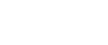Where, M= bending moment I = moment of inertia of the section about the bending axis.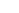=fibre stress at a distance ‘y’ from the centroidal/neutral axis. E = Young’s Modulus of the material of the beam. R = radius of curvature of the bent beam. If y is replaced by c, the distance to remotest element, then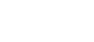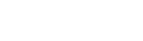Where, Z= section modulus and is given by, Z = I/c

## Types of  Bending Strain

### 1. Normal Strains

As a result of bending, somewhere between the top and bottom of the beam is a surface in which the longitudinal fibres do not change in length. This surface is called the neutral surface of the beam and its intersection with any cross-sectional plane is called the neutral axis of the cross-section. All the longitudinal fibres other than those in the neutral surface either lengthen or shorten, thereby creating longitudinal strains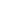.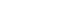Where k = curvature = 1/R This equation shows longitudinal strains are proportional to the curvature and that they vary linearly with the distance y from the neutral surface. This equation is derived from the geometry of the deformed beam and is independent of the properties of the material. The equation is valid irrespective of the stress-strain diagram of the material.

### 2. Transverse Strain

The axial strainsare accompanied by lateral or transverse strains due to the effect of Poisson’s ratio. Positive strains are accompanied by negative transverse strains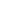.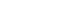Where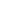is the Poisson’s Ratio. As a result of these strains, the shape of the cross-section change. For example, let us study the case of a beam of rectangular cross-section subjected to pure bending so as to induce tension at the top and compression at the bottom. The sides of the rectangular cross-section become inclined to each other. The top surface becomes saddle shaped. If the longitudinal curvature in the xy plane is considered positive, then the transverse curvature in the yz plane is negative. All planes of the beam that were initially parallel to neutral surface develop antiplastic curvature.

## Applicability of Flexure Formula

The normal stresses determined from flexure formula concern pure bending, which means no shear forces act on the cross-section. In case of non-uniform bending the presence of shear forces produces warping or put of place distortion of the cross-section, thus, a section that is plane before bending is no longer plane after bending. Warping due to shear greatly complicates the behaviour of the beam, but more elaborate analysis shows that the normal stresses calculated from the flexure formula are not significantly altered by the presence of the shear stresses and the associated warping. Thus use of the theory of pure bending for calculating normal stresses in cases of non-uniform bending is considered justified.